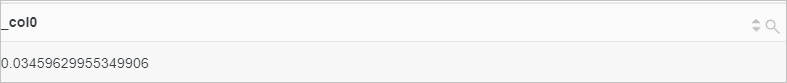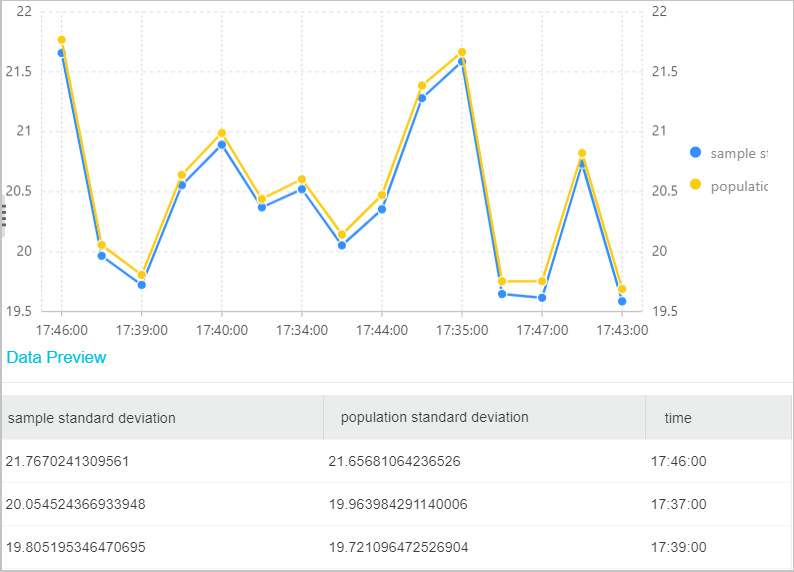This topic describes the syntax of mathematical statistics functions and provides usage examples.

## Basic syntax

Function Description
corr(key1, key2) Calculates the correlation between two specific columns.

Valid values: 0 to 1.

covar_pop(key1, key2) Calculates the population covariance of two specific columns.
covar_samp(key1, key2) Calculates the sample covariance of two specific columns.
regr_intercept(key1, key2) Returns the linear regression intercept of input values. key1 is the dependent value and key2 is the independent value.
regr_slope(key1, key2) Returns the linear regression slope of input values. key1 is the dependent value and key2 is the independent value.
stddev(key) Calculates the sample standard deviation of the key column. This function is equivalent to the stddev_samp function.
stddev_samp(key) Calculates the sample standard deviation of the key column.
stddev_pop(key) Returns the population standard deviation of the key column.
variance(key) Calculates the sample variance of the key column. This function is equivalent to the var_samp function.
var_samp(key) Calculates the sample variance of the key column.
var_pop(key) Calculates the population variance of the key column.

## Examples

• Example 1: Return the correlation of two specific columns.
• Query statement
``* | SELECT corr(request_length, request_time)``
• Query and analysis results• Example 2: Query the sample standard deviation and population standard deviation of pre-tax income.
• Query statement
``* | SELECT stddev(PretaxGrossAmount) as "sample standard deviation", stddev_pop(PretaxGrossAmount) as "population standard deviation", time_series(__time__, '1m', '%H:% I:%s', '0') AS time GROUP BY time``
• Query and analysis results